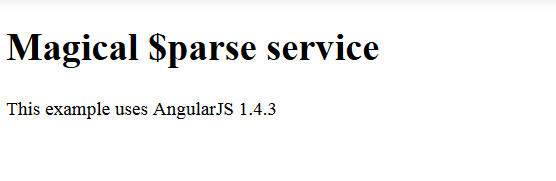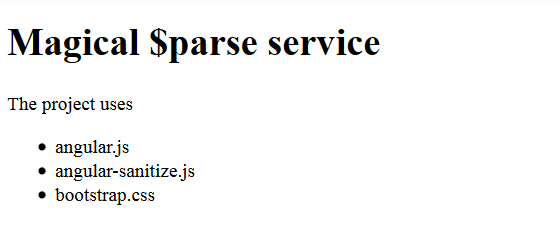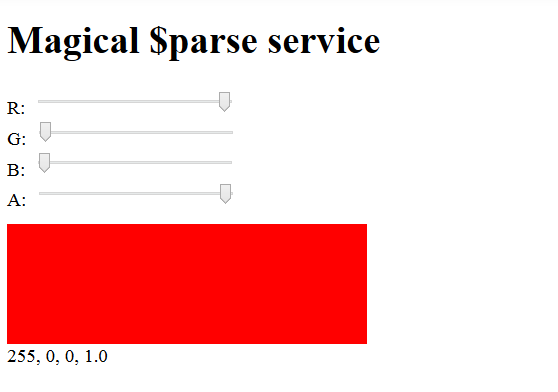Angular.js

# Magic \$parse service in AngularJS

AngularJS has a useful, but less documented \$parse service which is shortly described in the Angular’s docu as “converts Angular expression into a function”. In this post, I will explain what this description means and show explicit and implicit usages of \$parse. Furthermore, we will see the differences to the Angular’s \$interpolate service and \$eval method.

Implicitly used \$parse

The \$parse compiles an expression to a function which can be then invoked with a context and locals in order to retrieve the expression’s value. We can imagine this function as a pre-compiled expression. The first parameter is context – this is an object any expressions embedded in the strings are evaluated against (typically a scope object). The second parameter is locals – this is an JavaScript object with local variables, useful for overriding values in context. I’ve created a simple plunker to demonstrate the simple usage.

```<!DOCTYPE html>
<html>
<meta charset="utf-8" />
<meta http-equiv="X-UA-Compatible" content="IE=edge" />
<body ng-app="app">
<h1>Magical \$parse service</h1>

<div ng-controller="ParseController as vm">{{vm.parsedMsg}}</div>

<script src="https://code.angularjs.org/1.4.3/angular.js"></script>
<script src="app.js"></script>
</body>
</html>```

In the HTML we output the controller’s variable parsedMsg which is created in the app.js as follows:

```(function() {
angular.module('app', []).controller('ParseController', ParseController);

function ParseController(\$scope, \$parse) {
this.libs = {};
this.libs.angular = {
version: '1.4.3'
};

var template = \$parse("'This example uses AngularJS ' + libs.angular.version");
this.parsedMsg = template(this);
}
})();```

As you can see, the expression ‘This example uses AngularJS ‘ + libs.angular.version gets parsed and assigned to the variable template. The template is a function which is invoked with
this object as context. The this object containes the value of libs.angular.version (nested objects). This value is used when evaluating the pre-compiled expression. The result is saved in
this.parsedMsg and shown in the HTML.It is important to understand that \$parse can be invoked once and its result (pre-compiled expression) can be used multiple times with different context (and locals). The next plunker demonstrates the usage of just one pre-compiled expression with different locales. The HTML part:

```<!DOCTYPE html>
<html>
<meta charset="utf-8" />
<meta http-equiv="X-UA-Compatible" content="IE=edge" />
<body ng-app="app">
<h1>Magical \$parse service</h1>

<div ng-controller="ParseController as vm" ng-bind-html="vm.parsedMsg"></div>

<script src="https://code.angularjs.org/1.4.3/angular.js"></script>
<script src="https://code.angularjs.org/1.4.3/angular-sanitize.js"></script>
<script src="app.js"></script>
</body>
</html>```

The JavaScript part:

```(function() {
angular.module('app', ["ngSanitize"]).controller('ParseController', ParseController);

function ParseController(\$scope, \$parse) {
this.libs = ['angular.js', 'angular-sanitize.js', 'bootstrap.css'];

var template = \$parse("libs[i]")

var output = '';
for (var i = 0; i < this.libs.length; i++) {
output = output + '<li>' + template(this, {i: i}) + '</li>';
}

this.parsedMsg = 'The project uses <ul>' + output + '</ul>';
}
})();```

As you can see we pass {i: i} repeatedly as the second parameter, so that the expression libs[i] is always evaluated with the current value
i. That means libslibs, etc. The result looks like as follows:There are some cool things you can do with \$parse. I would like to only list three of them.

1) If the expression is assignable, the returned function (pre-compiled expression) will have an assign property. The assign property is a function that can be used to change the expression value on the given context, e.g. \$scope. Example:

```
\$parse('name').assign(\$scope, 'name2');
```

In the example, the value name on the \$scope has been changed to name2.

2) If we have an object with properties that might be null, no JS errors will be thrown when using \$parse. Instead of that, the result is set to undefined. In the example above, we had the property libs.angular.version. The result of

```
var version = \$parse('libs.angular.version')(this);
```

is version = 1.4.3. If we would use a non exisiting property in the expression, e.g.

```
var version = \$parse('libs.ember.version')(this);
```

the result would be version = undefined. AngularJS doesn’t throw an exception.

3) Theoretically you can send any logic from backend to the client as string and evaluate the parsed string on the client. Example:

```var backendString = 'sub(add(a, 1), b)';

var math = {
add: function(a, b) {return a + b;},
sub: function(a, b) {return a - b;}
};

var data = {
a: 5,
b: 2
};

var result = \$parse(backendString)(math, data); // 4```

Explicitly used \$parse

AngularJS also uses \$parse internally. The next example was taken from a book and slightly modified by me. It demonstrates a color picker with four sliders (three for colors and one for opacity).The whole code: the HTML page, the color-picker directive and app.js are demonstrated in my last plunker. The page uses the color-picker directive:

```<!DOCTYPE html>
<html>
<meta charset="utf-8" />
<meta content="IE=edge" http-equiv="X-UA-Compatible" />
<body ng-app="app">
<h1>Magical \$parse service</h1>

<div ng-controller="MainController as vm">
<color-picker init-r="255"
init-g="0"
init-b="0"
init-a="1.0"
on-change="vm.onColorChange(r,g,b,a)">
</color-picker>
</div>

<script src="https://code.angularjs.org/1.4.3/angular.js"></script>
<script src="app.js"></script>
</body>
</html>```

The directive’s template colorPickerTemplate.html renders four input sliders, a live preview and current colors and opacity.

```R:<input type="range"
min="0" max="255" step="1"
ng-model="r">
<br>
G:<input type="range"
min="0" max="255" step="1"
ng-model="g">
<br>
B:<input type="range"
min="0" max="255" step="1"
ng-model="b">
<br>
A:<input type="range"
min="0" max="1" step="0.01"
ng-model="a">

<div style="width: 300px; height: 100px; margin-top:10px;
background-color: rgba({{ r }}, {{ g }}, {{ b }}, {{ a }});">
</div>
{{ r }}, {{ g }}, {{ b }}, {{ a }}```

The JavaScript part links all together. Whenever a color or opacity is changed, the Angular’s \$log service logs current colors and opacity in the browser dev. console. The current colors and opacity are also shown permanently as mentioned above.

```(function() {
angular.module('app', [])
.controller('MainController', MainController)
.directive('colorPicker', colorPickerDirective);

function MainController(\$log) {
this.onColorChange = function(r, g, b, a) {
\$log.log('onColorChange', r, g, b, a);
};
}

function colorPickerDirective() {
return {
scope: {
r:        '@initR',
g:        '@initG',
b:        '@initB',
a:        '@initA',
onChange: '&'
},
restrict: 'E',
templateUrl: 'colorPickerTemplate.html',
['r', 'g', 'b', 'a'].forEach(function(value) {
scope.\$watch(value, function(newValue, oldValue) {
if (newValue !== oldValue) {
if (angular.isFunction(scope.onChange)) {
scope.onChange({
'r': scope.r,
'g': scope.g,
'b': scope.b,
'a': scope.a
});
}
}
});
});
}
};
}
})();```

But stop, where is the magic \$parse here? The directive defines a parameter for an onchange callback via onChange: ‘&’. Behind the scenes, AngularJS parses the passed onchange string, in this case vm.onColorChange(r,g,b,a), and creates a function (discussed pre-compiled expression) which can be invoked with the desired context and locales. In the directive, we invoke this function with current values for colors r, g, b and opacity a.

```scope.onChange({
'r': scope.r,
'g': scope.g,
'b': scope.b,
'a': scope.a
});```

Relation to \$interpolate and \$eval

\$eval is a method on a scope. It executes an expression on the current scope and returns the result. Example:

```scope.a = 1;
scope.b = 2;

scope.\$eval('a+b'); // result is 3```

Internally, it uses \$parse for their part.

```\$eval: function(expr, locals) {
return \$parse(expr)(this, locals);
}```

Note, that the \$eval method can not precompile expressions and be used repeatedly. The \$parse, in contrast, can be invoked once in one place and its result can then be used repeatedly elsewhere. We have seen that in the second plunker. Here is another simple example to emphasize the difference to \$eval:

```var parsedExpression = \$parse(...);

var scope1 = \$scope.\$new();
var scope2 = \$scope.\$new();

...

var result1 = parsedExpression(scope1);
var result2 = parsedExpression(scope2);```

There is another high level service – \$interpolate. By means of \$interpolate you can do on-the-fly templating like well-known template engines Handlebars or Hogan do that. ES6 has similar template strings too. But take a look at one \$interpolate example.

```(function() {
angular.module('app', []).controller('InterpolateController', InterpolateController);

function InterpolateController(\$scope, \$interpolate) {

var bookData1 = {title: 'PrimeFaces Cookbook', publisher: 'Packt'};
var bookData2 = {title: 'Spring in Action', publisher: 'Manning'};

// execute the function "template" and pass it data objects to force interpolation
var bookInfo1 = template(bookData1);
var bookInfo2 = template(bookData2);

// the result:
}
})();```

As you can see, the \$interpolate service takes a string and returns a function which is a compiled template. Expressions in the passed string may have all allowable Angular’s expression syntax. E.g. a pipe with filter as in the example {{publisher | uppercase}}. The function can be called again and again with different data objects. The \$interpolate service uses \$parse internally to evaluate individual expressions against the scope. In this regard, the \$interpolate service is normally used as a string-based template language for strings containing multiple expressions. In contrast to \$parse, the \$interpolate is read only.

 Reference: Magic parse service in AngularJS from our WCG partner Oleg Varaksin at the Thoughts on software development blog.

## Do you want to know how to develop your skillset to become a Web Rockstar?

### Oleg Varaksin

Subscribe
Notify ofThis site uses Akismet to reduce spam. Learn how your comment data is processed.

1 Comment
Inline Feedbacks